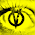## Monday, February 18, 2008

### Memory efficient Brainfuck tables

Here is a nice way to implement an indexed table in Brainfuck, using only n+4 memory for n table cells. I will soon release a new version of FBF that uses this implementation instead of the old one which used 2*n+3. (Well maybe not... I am bored...)

The table has the following structure:

0 I I D (0) (1) (2) (3) ...
^

For a table with n cells you will need n+4 cells on the Brainfuck array.
( n <= cell size) We start from where the ^ points. The first cell should be empty (zero). The second and third cells contain the index that we want to write to or read from. The fourth cell contains the data that we want to store in the table. If don't want to read from the table then we should leave this cell empty and it will later carry the read data. The next cells (0) (1) ... (n-1) are the actual data stored in the table. We'll call the I I D part the "head". It works like this: Move the head to the right place: (the head moves to the right by moving the data of the next cell to the head's preceding empty cell, then moving each of the I I D cells one place to the right creating a new empty preceding cell.)

We will move the head to the right to the correct index:
>[
>>>[-<<<<+>>>>]
<
If we want to write something then we should carry the D cell too: [->+<]
<[->+<]
<[->+<]
>-]

>>>[-<+<<+>>>]<<<[->>>+<<<]>
Or we may write:
>>>[-]<[->+<]<

Now we will return the head to it's initial position:
[
[-<+>]
If we have read something then we need to carry the D cell too: >[-<+>]<
<<<[->>>>+<<<<]
>>-]
<<

After a successful read the I I cells should be empty while the D cell will hold the read data.
After a successful write the I I D cell should be empty.

The pointer ends at the some place where it was before we used the algorithm, that is to the left if the first I cell.

Here is the code uncommented:

Write:
>[>>>[-<<<<+>>>>]<[->+<]<[->+<]<[->+<]>-]
>>>[-]<[->+<]<
[[-<+>]<<<[->>>>+<<<<]>>-]<<

>[>>>[-<<<<+>>>>]<<[->+<]<[->+<]>-]
>>>[-<+<<+>>>]<<<[->>>+<<<]>
[[-<+>]>[-<+>]<<<<[->>>>+<<<<]>>-]<<

1.This is really great but a more in-depth explanation couldn't hurt.

1.Thank you. Yeah I could give some more details on this one. I'll put it in my to-do list to rewrite it but be warned that my todo list is way too long.

But if you really want help understanding this code I could explain it over Discord (voice chat) if you are interested. I'm Tritonio#6813 there. If you cannot add me for whatever reason, just comment here again and I can find another way to connect with you. Chatting is not boring in contrast to rewriting a post I made 10+ years ago. ;-)

2.Hey, just thought I should let you know that these algorithms are really awesome! I could never figure out how to do something like do > or < n times so this is really helpful :)

1.Thanks! :-) How did you find this page btw? I got two comments within a few days and the post never had a comment for 10 years, and back then blogs were actually popular.

2.Personally I found your blog via https://github.com/arthaud/brainfuck-cpu